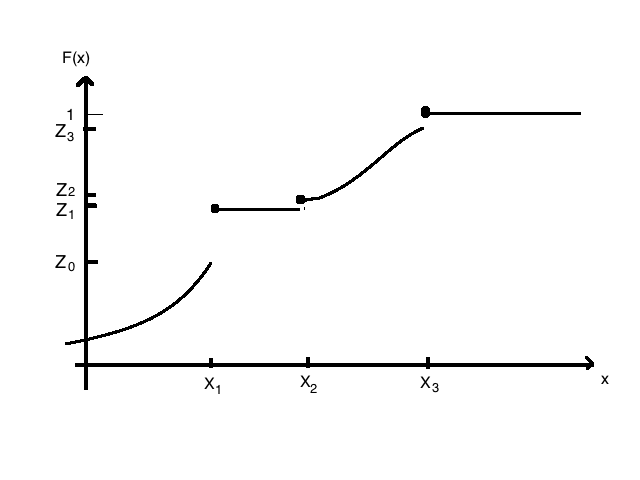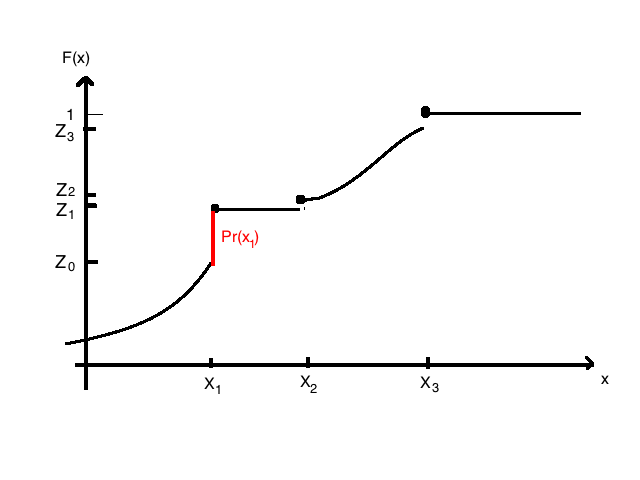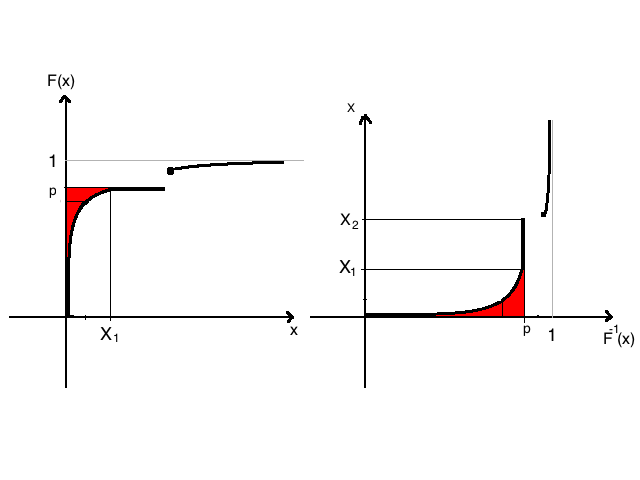Abstract: 本文介绍描述随机变量分布的另一种工具，累积分布函数，CDF
Keywords: Cumulative Distribution Function，Quantial

# 累积分布函数

## 累积分布函数的定义和基本性质 Definition and Basic Properties

Definition (Cumulative) Distribution Function :The distribution function or cumulative distribution function(abbreviated c.d.f) $F$ of a random variable $X$ is the function:
$$F(x)=Pr(X\leq x)\quad \text{for} \quad -\infty<x<+\infty$$

$$F(x)= \begin{cases} 0 &\text{for} &x<0\\ 1-p &\text{for}& 0\leq x<1\\ 1 &\text{for}&x\geq 1 \end{cases}$$

### 性质1：不减性 Nondecreasing

The function $F(x)$ is nondecreasing as $x$ increases;that is,if $x_1<x_2$ then $F(x_1)\leq F(x_2)$

$$\text{for }x_1\leq x_2\\ {x:x<x_1}\subset {x:x<x_2}$$

$$Pr({x:x<x_1})\leq Pr( {x:x<x_2})$$
Q.E.D.

### 性质2：有限 Limits

Limits at $\pm\infty$ $lim_{x\to -\infty}F(x)=0$ and $lim_{x\to \infty}F(x)=1$

$$\text{for }x_1\leq x_2\\ {x:x<x_1}\subset {x:x<x_2}$$

$Pr({x:x<x_1})=1 \quad \text{for } x_1=+\infty$

$Pr({x:x<x_2})=0 \quad \text{for } x_2=-\infty$

c.d.f不需要有连续性，如图：$$F(x^{-})=lim_{y\to x,y<x}F(y)\\ F(x^{+})=lim_{y\to x,y>x}F(y)$$

### 性质3：向右连续 Continuity from the Right

Continuity from the Right.A c.d.f. is always continuous from the right: that is,$F(x)=F(x^{+})$ at every point $x$

## 从分布函数中确定概率 Determining Probabilities from the Distribution Function

### T1： $Pr(X>x) = 1 - F(x)$

Theorem: For every value $x$,
$$Pr(X>x) = 1 - F(x)$$
QED

### T2:$Pr(x_1<X\leq x_2)=F(x_2)-F(x_1)$

Theorem :For all value $x_1$ and $x_2$ such that $x_1<x_2$ :
$$Pr(x_1<X\leq x_2)=F(x_2)-F(x_1)$$

$$B=A^{c}\cap C$$

$$Pr(B)=Pr(C)-Pr(A\cap C)\\ \text{for: }A=A\cap C\\ Pr(B)=Pr(C)-Pr(A)\\ Pr(x_1<X\leq x_2)=F(x_2)-F(x_1)$$
QED

### T3:$Pr(X<x)=F(x^{-})$

Theorem For each value $x$
$$Pr(X<x)=F(x^{-})$$

### T4:$Pr(X=x)=F(x)-F(x^{-})$

Theorem For every value $x$
$$Pr(X=x)=F(x)-F(x^{-})$$

$$Pr(X=x)=Pr(X\leq x)-Pr(X<x)$$

$$F(x)=Pr(X\leq x)$$## 离散分布的累积函数 The c.d.f. of a Discrete Distribution

1. $F(x)$ will have a jump of magnitude $f(x_i)$ at each possible valuee $x_i$ of $X$
2. $F(x)$ will be constant between every pair of successive jump
3. The distribution of a discrete random variable $X$ can be represented equally well by either the p.f. or the c.d.f. of $X$

## 连续分布的累积函数 The c.d.f. of a Continuous Distribution

Theorem Let X have a continuous distribution, and let f(x) and F(x) denote its p.d.f and the c.d.f. respectively.Then $F$ is continuous at every $x$:
$$F(x)=\int^{x}_{-\infty}f(t)dt$$
and
$$\frac{dF(x)}{dx}=f(x)$$
at all x such that f is continuous.

## 分位数函数 The Quantile Function

Definition Quantiles/Percentiles: Let $X$ be a random variable with c.d.f. $F$ .For each $p$ strictly between $0$ and $1$ ,define $F^{-1}(p)$ to be the smallest value $x$ such that $F(x)\geq p$ .Then $F^{-1}(p)$ is called the $p$ quantile of $X$ or the $100p$ percentile of $X$ .The function $F^{-1}$ defined here on the open interval $(0,1)$ is called the quantile function of $X$### 中位数 Median quartiles

Definition Median/Quantiles.The $\frac{1}{2}$ quantile or the 50th percentile of a distribution is called its median.The $\frac{1}{4}$ quantile or 25th percentile is the lower quartile.The $\frac{3}{4}$ quantile or 75th percentile is called upper quartile.

0%